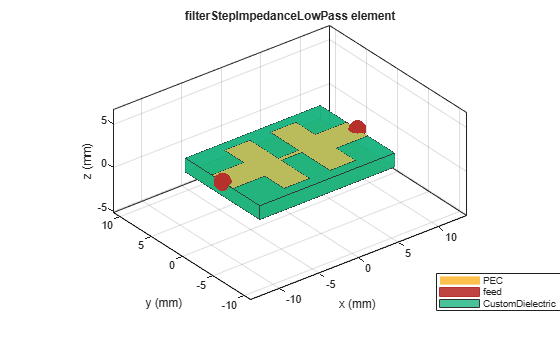# filterStepImpedanceLowPass

Create stepped impedance lowpass filter in microstrip form

Since R2021b

## Description

Use the `filterStepImpedanceLowPass` object to create a stepped impedance lowpass filter in microstrip form.The stepped impedance lowpass microstrip filters have a cascaded structure of alternating high- and low-impedance transmission lines. These lines are considerably shorter in length than the design wavelength and act as semi-lumped elements. The high-impedance lines act as series inductors, and the low-impedance lines act as shunt capacitors. This filter structure realizes a Pi LC ladder type of a lowpass filter. You can control the impedance by adjusting the width of the strip. This filter is used in radar, satellite, and terrestrial communications and in electronic counter-measure applications.

## Creation

### Syntax

``filter = filterStepImpedanceLowPass``
``filter = filterStepImpedanceLowPass(Name=Value)``

### Description

example

````filter = filterStepImpedanceLowPass` creates a default stepped impedance lowpass filter with default property values for a cutoff frequency 5 GHz. ```

example

````filter = filterStepImpedanceLowPass(Name=Value)` sets Properties using one or more name-value arguments. For example, `filterStepImpedanceLowPass(FilterOrder=10)` creates a tenth-order stepped impedance lowpass filter. Properties not specified retain their default values```

## Properties

expand all

Filter order, specified as a positive scalar. The minimum filter order you can specify is 3 and the maximum order is 11.

Example: ```filter = filterStepImpedanceLowPass(FilterOrder=5)```

Data Types: `double`

Length of the input and output lines in meters, specified as a positive scalar.

Example: ```filter = filterStepImpedanceLowPass(PortLineLength=0.0553)```

Data Types: `double`

Width of the input and output lines in meters, specified as a positive scalar.

Example: ```filter = filterStepImpedanceLowPass(PortLineWidth=0.0087)```

Data Types: `double`

Width of the low-impedance line in meters, specified as a positive scalar.

Example: ```filter = filterStepImpedanceLowPass(LowZLineWidth=0.0553)```

Data Types: `double`

Width of the high-impedance line in meters, specified as a positive scalar.

Example: ```filter = filterStepImpedanceLowPass(HighZLineWidth=0.0553)```

Data Types: `double`

Length of the low-impedance line in meters, specified as a positive scalar.

Example: ```filter = filterStepImpedanceLowPass(LowZLineLength=0.0553)```

Data Types: `double`

Length of the high-impedance line in meters, specified as a positive scalar.

Example: ```filter = filterStepImpedanceLowPass(HighZLineWidth=0.0553)```

Data Types: `double`

Height of the filter from the ground plane in meters, specified as a positive scalar.

Example: ```filter = filterStepImpedanceLowPass(Height=0.020)```

Data Types: `double`

Width of the ground plane in meters, specified as a positive scalar.

Example: ```filter = filterStepImpedanceLowPass(GroundPlaneWidth=0.013)```

Data Types: `double`

Type of dielectric material used as a substrate, specified as a `dielectric` object. The dielectric material in a `filterStepImpedanceLowPass` object with default properties have the following properties:

• `Name``{'CustomDielectric'}`

• `EpsilonR``3.7`

• `LossTangent``0.001`

• `Thickness``1.6e-3`

Example: `d = dielectric("FR4");` `filter = filterStepImpedanceLowPass(Substrate=d)`

Data Types: `string` | `char`

Type of metal used in the conducting layers, specified as a `metal` object. The type of metal in a `filterStepImpedanceLowPass` object with default properties is PEC.

Example: `m = metal("Copper");` `filter = filterStepImpedanceLowPass(Conductor=m)`

Data Types: `string` | `char`

## Object Functions

 `charge` Calculate and plot charge distribution `current` Calculate and plot current distribution `design` Design stepped impedance low pass filter around desired cut-off frequency `feedCurrent` Calculate current at feed port `getZ0` Calculate characteristic impedance of transmission line `layout` Plot all metal layers and board shape `mesh` Change and view mesh properties of metal or dielectric in PCB component `shapes` Extract all metal layer shapes of PCB component `show` Display PCB component structure or PCB shape `sparameters` Calculate S-parameters for RF PCB objects

## Examples

collapse all

Create and view a default stepped impedance lowpass filter.

`steppedfilter = filterStepImpedanceLowPass`
```steppedfilter = filterStepImpedanceLowPass with properties: FilterOrder: 3 PortLineWidth: 0.0034 PortLineLength: 0.0040 HighZLineWidth: 5.0000e-04 LowZLineWidth: 0.0096 HighZLineLength: 0.0026 LowZLineLength: 0.0032 Height: 0.0016 GroundPlaneWidth: 0.0120 Substrate: [1x1 dielectric] Conductor: [1x1 metal] ```
`show(steppedfilter)`Create and view a stepped impedance lowpass filter with a multilayer dielectric substrate.

```sub = dielectric("FR4","Teflon"); sub.Thickness =[0.003 0.001]; steppedfilter = filterStepImpedanceLowPass; steppedfilter.Height = 0.003; steppedfilter.Substrate = sub; figure show(steppedfilter)```Plot the charge and current on the filter at 5 GHz.

```figure charge(steppedfilter,5e9)``````figure current(steppedfilter,5e9)````info(steppedfilter)`
```ans = struct with fields: IsSolved: "true" IsMeshed: "true" MeshingMode: "auto" HasSubstrate: "true" HasLoad: "false" PortFrequency: [] MemoryEstimate: "790 MB" ```

 Pozar, David M. Microwave Engineering. 4th ed. Hoboken, NJ: Wiley, 2012.

 Garvansh, Abhay, Singh Kushwaha, Navita Singh, and Arun Kumar. “Implementation of Stepped Impedance Low Pass Microstrip Line Filter for Wireless Communication.” International Journal of Advanced Research in Computer and Communication Engineering 3, no. 7 (July 2014): 7608–10.

 Maity, Budhadeb. “Stepped Impedance Low Pass Filter Using Microstrip Line for C-Band Wireless Communication.” In 2016 International Conference on Computer Communication and Informatics (ICCCI), 1–4. Coimbatore, India: IEEE, 2016. https://doi.org/10.1109/ICCCI.2016.7480008.